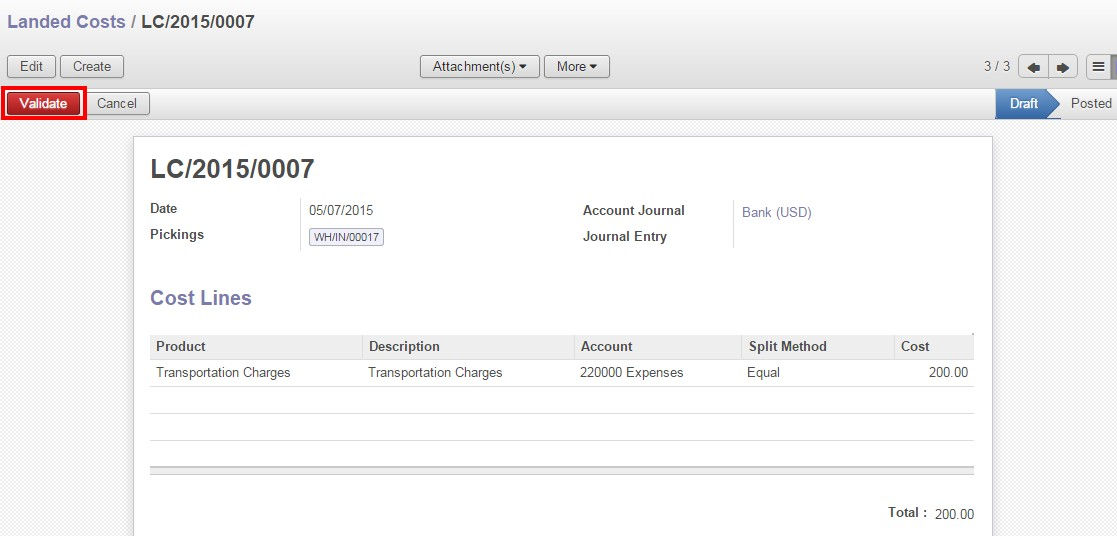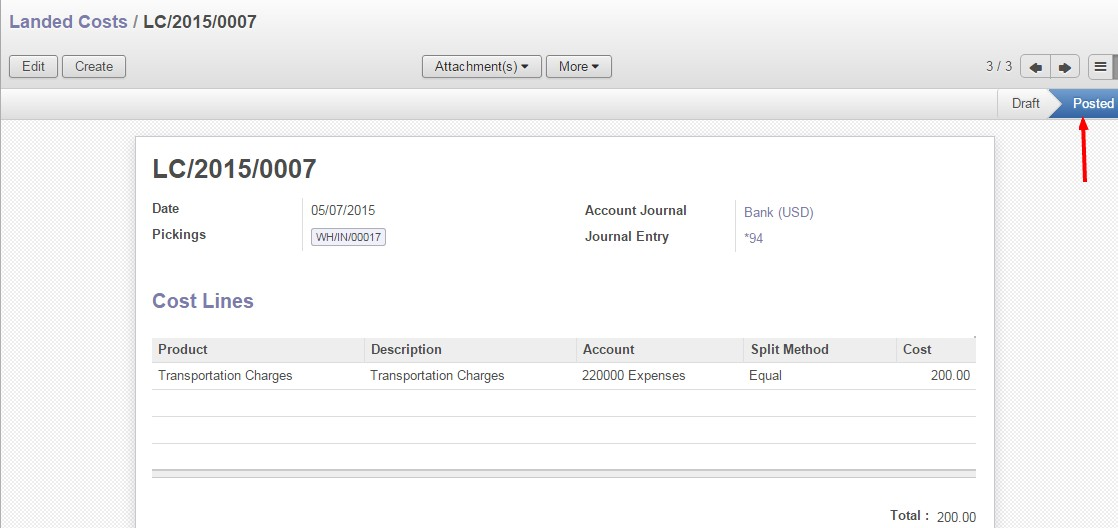# Calculating landed Cost in ERP

This blog explains how purchase managers, material accountants, and finance professionals can use ERPinCloud to calculate the landing cost.

What is Landed Cost :- Let us once again explain you about Landing Cost

The total cost of a landed shipment including freight, insurance and other costs up to the port of destination. In Some instances, it may also include the customs duties and other taxes levied on incoming shipment.

Now, Let us see how to Configure Landed Cost in ERPinCloud:-

Go To ->>Setting ->> Configuration ->> Warehouse ->> Accounting ->> Check the checkbox “Calculated Landed Costs on Product”->> Click on apply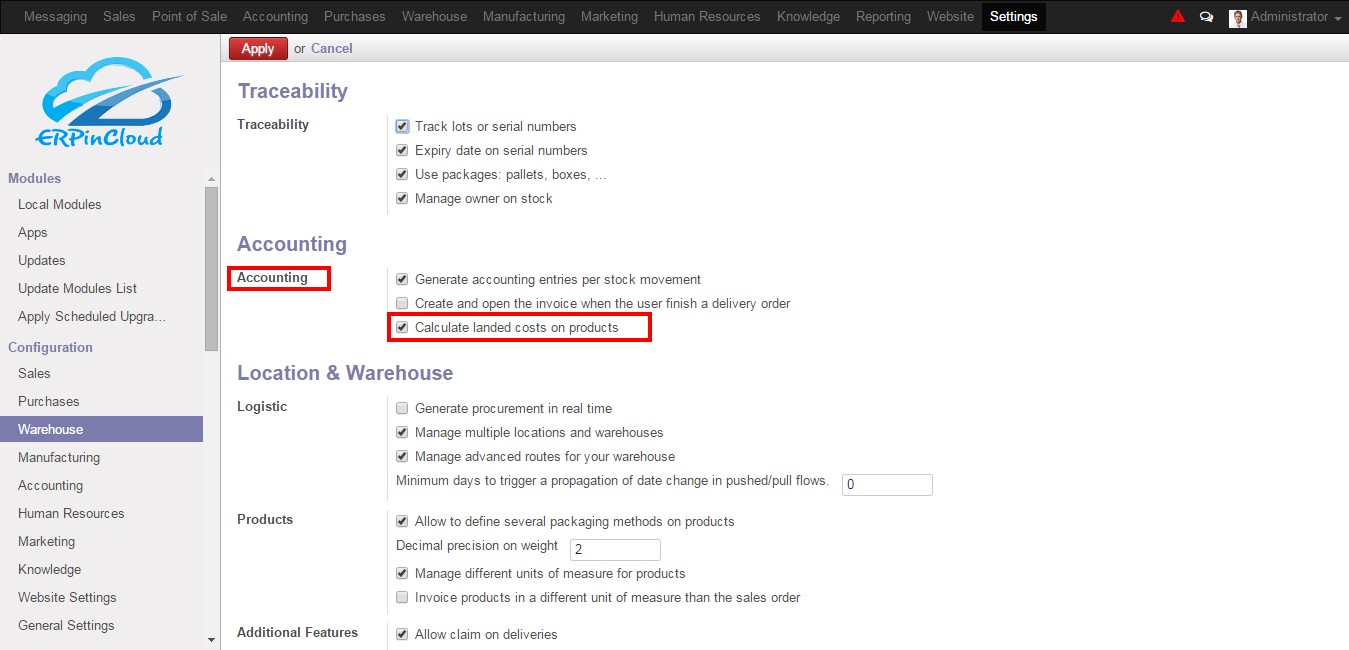Product Level Configuration:-

1. Go to ->> Warehouse ->> Product ->> Select a Product ->> Accounting ->> Select the Inventory Valuation ->> Real Time (automated)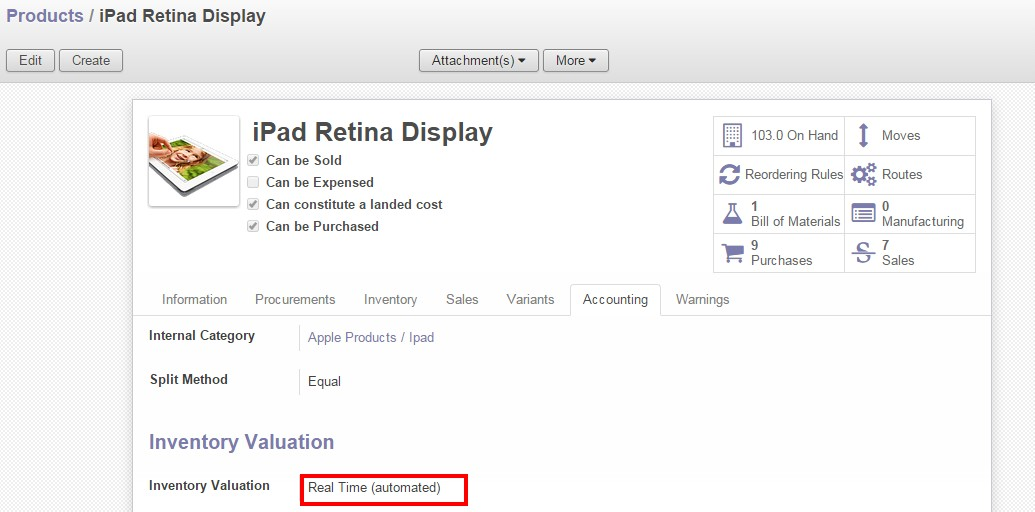2. In product select costing method as “Real Price”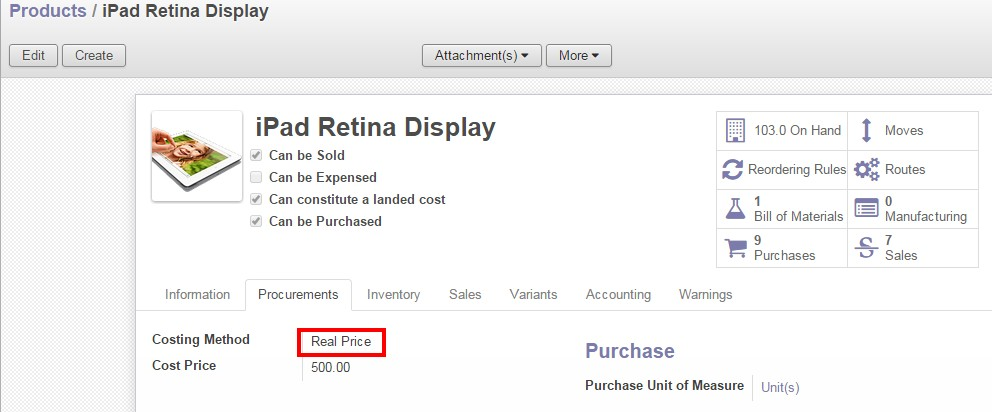3.  Check the checkbox “Can constitute a landed cost”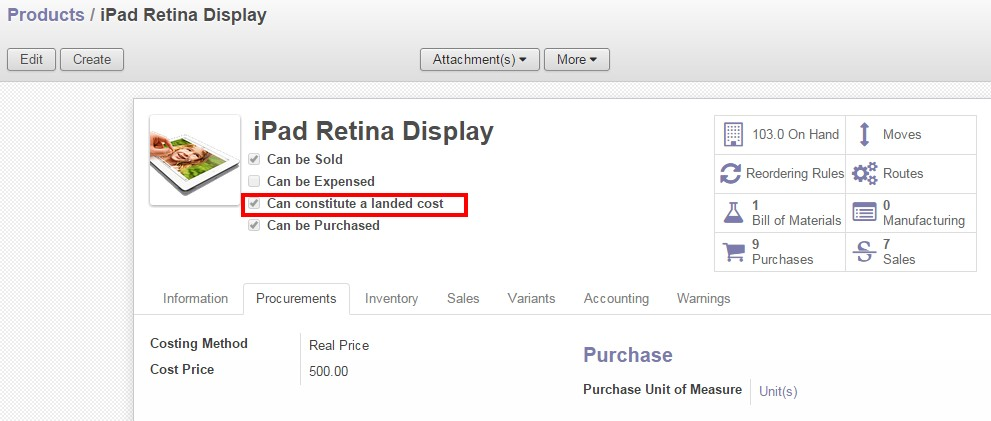Landed Cost Type Configuration:-

Go to ->> Warehouse ->> Configuration ->> Landed Cost Type ->> Create ->> Enter Product Name ->>  Select the Split Method ->> Select the Expense Account  ->> Select the Product Type ->> Save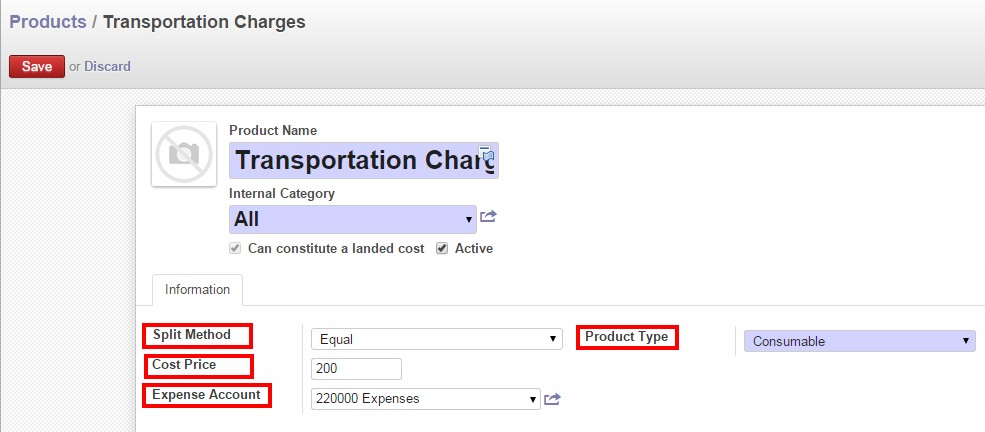Split Method:-

Equal: – Divides whole cost into equal

By Quantity: – Calculates cost according to quantity

By Current Cost Price: – Calculates cost according to cost price

By Weight: – Calculates cost according to weight

By Volume: – Calculates cost according to volume

Flow of Landed Cost:-

Step-1:-

a. Split Method-Equal:-

1. Go to ->> Warehouse ->> Landed Cost ->> Create ->> Fill Date, Account Journal & Pickings ->> Add an item ->> Select Split Method – >> Equal ->> Save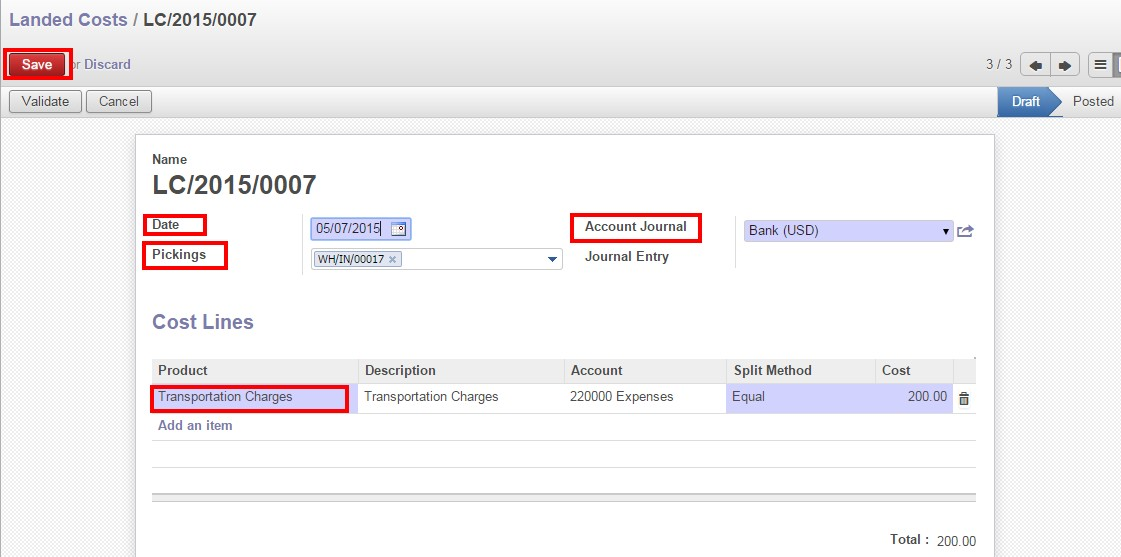Cost Price=200 (Defined in landed cost type-transportation charges)

2. Click on compute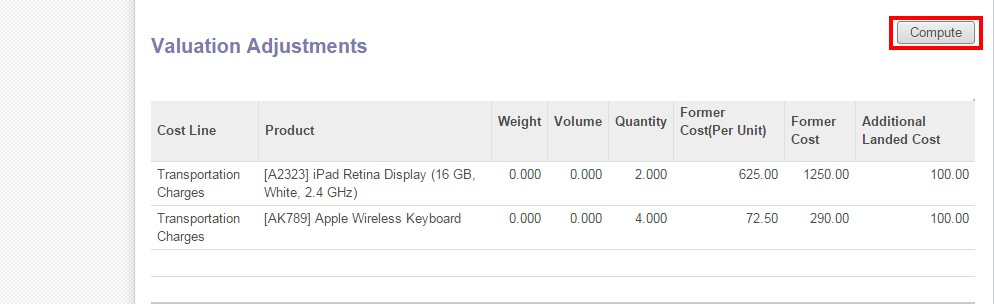After clicking on compute button the landed cost will get reflected according to the split method (in additional landed cost) in the valuation adjustments.

Calculation:-

Split Method-Equal

Cost price=200

Number of products=2

Formula:-Cost price/Number of products=200/2=100

b.  Split Method-By Quantity:-

1. Go to ->> Warehouse ->> Landed Cost ->> Create ->> Fill Date, Account Journal & Pickings ->> Add an item ->> Select Split Method – >> By Quantity ->> Save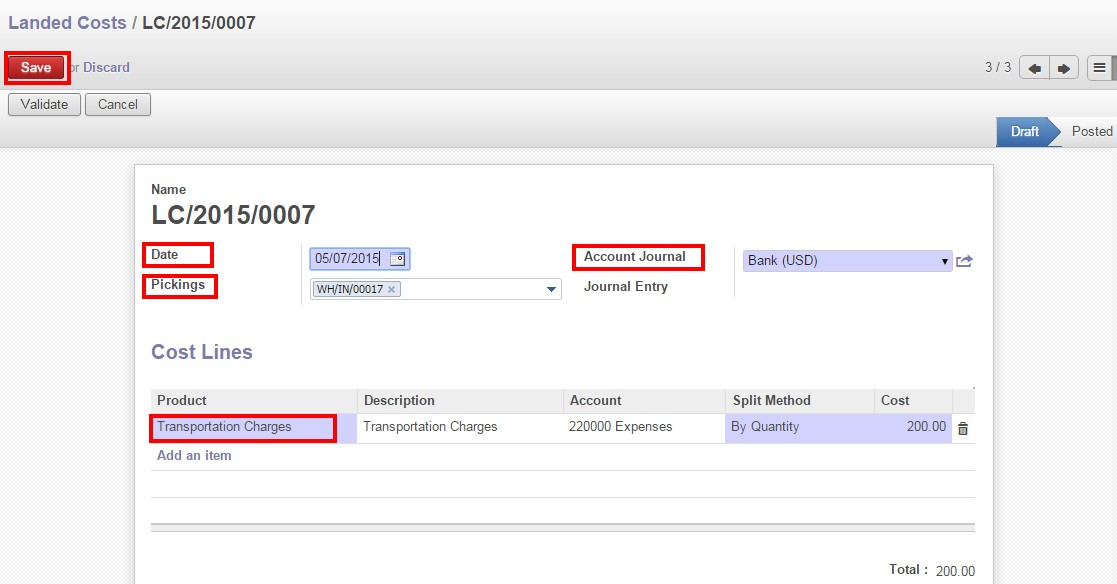2. Click on compute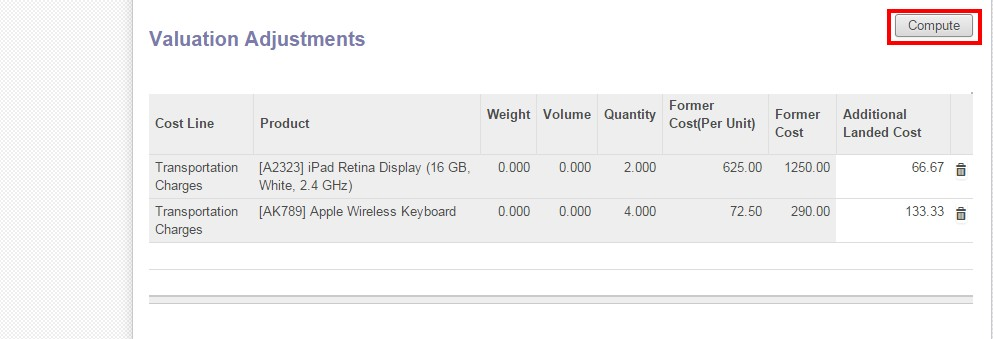After clicking on compute button the landed cost will get reflected according to the split method (in additional landed cost) in the valuation adjustments.

Calculation:-

Split Method-By Quantity

Cost price=200

Number of products=2

First Product [A2323]=2 quantities

Second Product [AK789]=4 quantities

Total number of quantities=6

Formula:- Cost per quantity=Cost price/Total number of quantities=200/6=33.33

Additional landed cost for the first product=33.33*2=66.66

Additional landed cost for the second product=33.33*4=133.32

c.  Split Method-By Current Cost Price

1. Go to ->> Warehouse ->> Landed Cost ->> Create ->> Fill Date, Account Journal & Pickings ->> Add an item ->> Select Split Method – >> By Current Cost Price ->> Save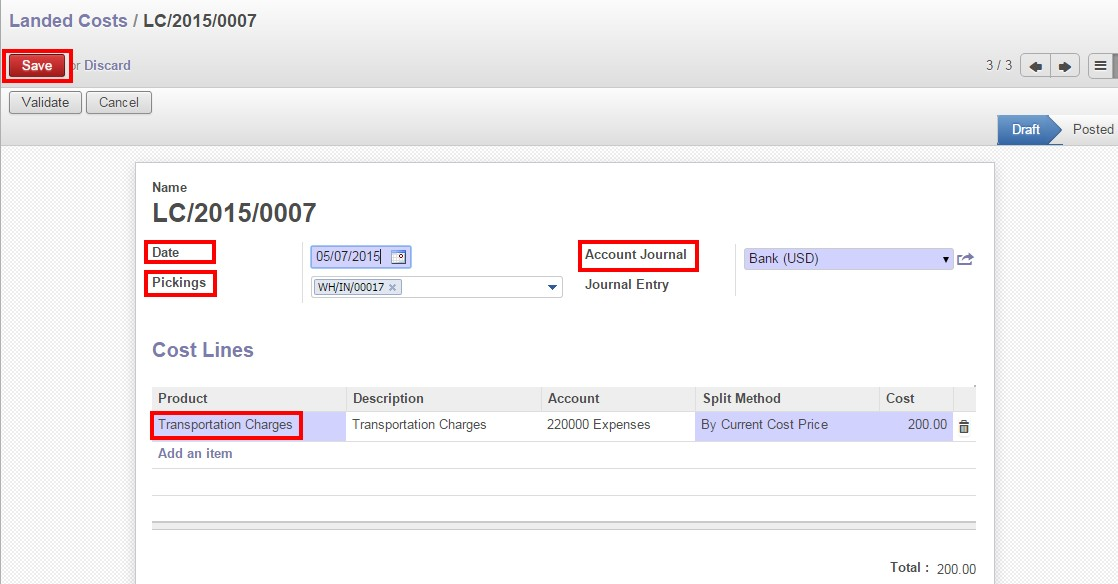2. Click on compute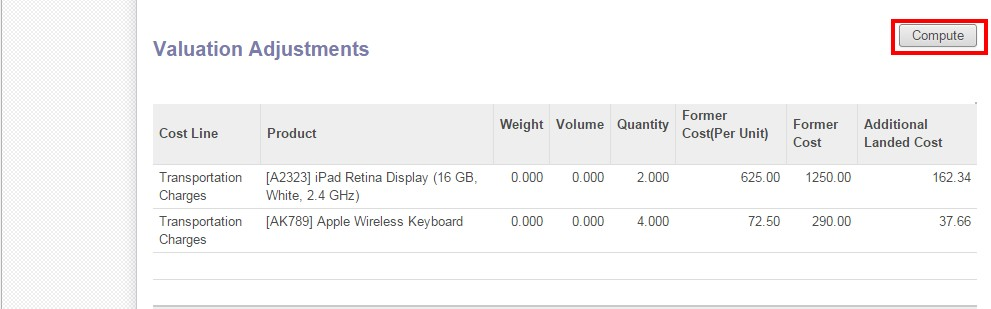After clicking on compute button the landed cost will get reflected according to the split method (in additional landed cost) in the valuation adjustments.

Calculation:-

Split Method-By Current Cost PriceCost price=200

First Product [A2323](former cost price)=1250

Second Product [AK789](former cost price)=290

Total former cost price=(1250+290)=1540

Formula:-

Additional landed cost for the first product=

(Former cost price/Total former cost price)*Cost price=(1250/1540)*200=162.33

Additional landed cost for the second product=

(Former cost price/Total former cost price)*Cost price=(290/1540)*200=37.66

d. Split Method-By Weight

1. Go to ->> Warehouse ->> Landed Cost ->> Create ->> Fill Date, Account Journal & Pickings ->> Add an item ->> Select Split Method – >> By Weight ->> Save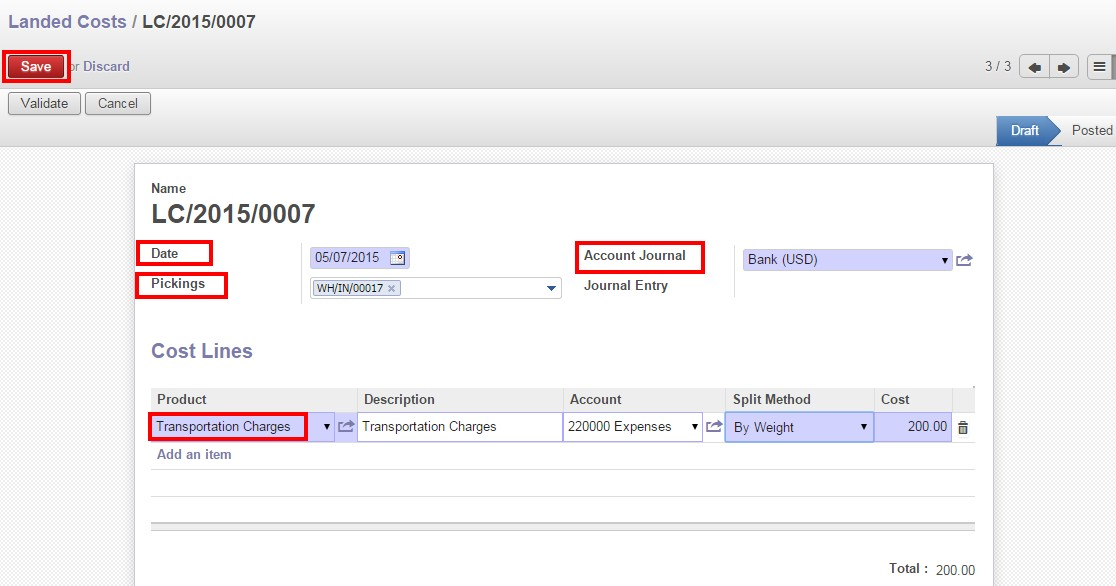2. Click on compute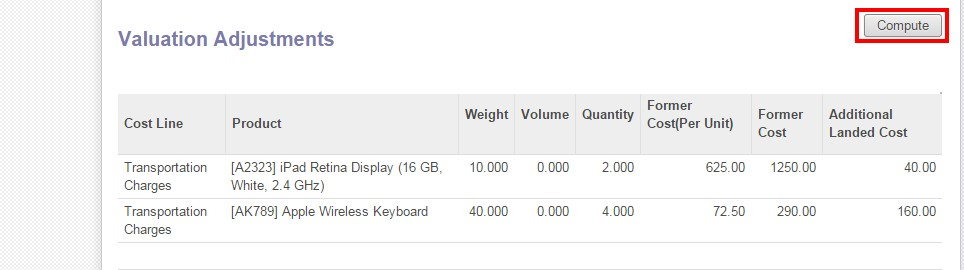After clicking on compute button the landed cost will get reflected according to the split method (in additional landed cost) in the valuation adjustments.

Calculation:-

Split Method-By Weight

Cost price=200

First Product [A2323]-weight=10

Second Product [AK789]-weight=40

Total weight of both products=(10+40)=50

Formula:-

Additional landed cost for the first product=

(Weight/ Total weight of both products)*Cost price=(10/50)*200=40

Additional landed cost for the second product=

(Weight / Total weight of both products)*Cost price=(40/50)*200=160

e. Split Method-By Volume

1. Go to ->> Warehouse ->> Landed Cost ->> Create ->> Fill Date, Account Journal & Pickings ->> Add an item ->> Select Split Method – >> By Volume ->> Save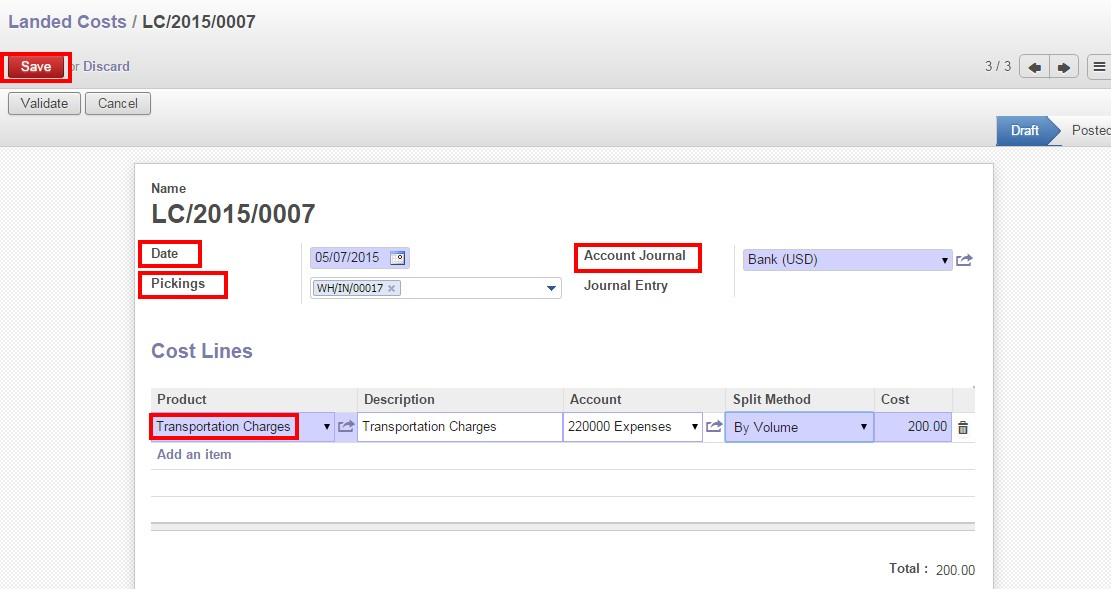2. Click on compute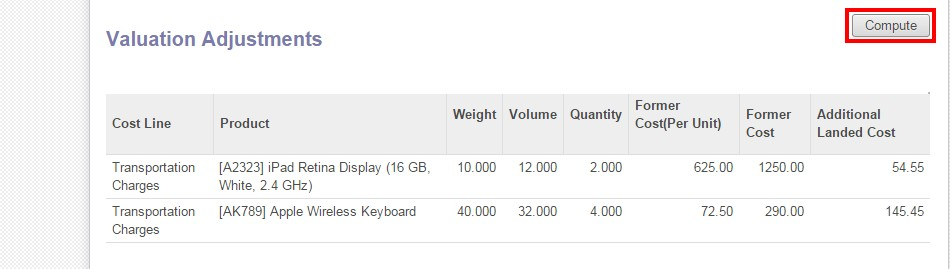After clicking on compute button the landed cost will get reflected according to the split method (in additional landed cost) in the valuation adjustments.

Calculation:-

Split Method-By Volume

Cost price=200

First Product [A2323]-volume=12

Second Product [AK789]-volume=32

Total weight of both products=(12+32)=44

Formula:-

Additional landed cost for the first product=

(Volume/ Total volume of both products)*Cost price=(12/44)*200=54.54

Additional landed cost for the second product=

(Volume/ Total volume of both products)*Cost price=(32/44)*200=145.45

Step-2:-

Click on Validate